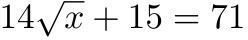Order of Operations Practice For Your Elementary School Child

Order of Operations PracticeIf your child is struggling with learning the order of operations, this lesson can help your child grasp the concept. This lesson provides order of operations practice that can help your child truly understand order of operations. Feel free to use these free worksheets on order of operations. Teach your child the old mantra, My Dear Aunt Sally, to remind them that it's multiplication, division, addition, and then subtraction.

Before you get started, you need to evaluate what grade level your child is currently functioning at. If your child is at the second grade level, you will want to start giving them problems that only involve addition and subtraction. If they are at the 3rd or 4th grade level, also give them multiplication and division. Around the 5th grade level, in addition to adding, subtracting, multiplying, and dividing, you need to introduce grouping symbols such as (parentheses), {braces}, and [brackets.] If you child is in middle school you can add exponents to the mix.

One strategy that I utilize for all my students when doing order of operations is to underline the part that they are working on, solve that part, then rewrite the problem based on what is left. So, let’s take a look at how you can do a lesson on order of operations with your child.

The first thing you need to do is introduce the order of operations. Remember to use the above suggestions on what part to work on based on your child’s grade level. Note that addition and subtraction can be reversed; they are done in the order of which comes first, and the same goes for multiplication and division. Here are example problems to do based on what level your child is working at. Keep the problems simple at first, and then increase the difficulty by making the numbers larger.

5 + 3 – 2 10 – 4 + 9 20 + 2 – 7

2 – 2 6 + 9 22 – 7

0 15 15

16 ÷ 4 + 8 – 2 x 3 3 x 5 – 9 ÷ 3 + 5

4 + 8 – 2 x 3 15 – 9 ÷ 3 + 5

4 + 8 – 6 15 – 3 + 5

12 – 6 15 – 8

6 7

Grouping Symbols (in addition to the other operations)

[3 + 9] x 2 – 7 {(5 – 4) + (6 x 2)}

12 x 2 – 7 {(5 – 4) + 12}

24 – 7 {1 + 12}

17 13

Exponents (You can do grouping symbols and exponents at the same time if you want, but I suggest introducing them separately

32 x 4 + (6 – 2 + 5)

32 x 4 + (4 + 5)

32 x 4 + 9

9 x 4 + 9

36 + 9

45

Here is a great game to play to help reinforce the order of operations. Write all of the operations on an index card. Shuffle them up, then give them to your child and tell them to put them in order. Another option is to only write the symbols on the cards and have your child put them in order as well.

After you play a game, it is time to start practicing. It is easy to create problems on your own, but there are a great number of Internet resources that provide free worksheets on the order of operations, so if you don’t have time to create them, print them off the Internet.

The key to helping your child succeed with this is to practice, practice, practice. However, do not overwhelm your child. These types of problems do take a little time to complete, so I suggest doing no more than 10 problems at a time.

If you are a teacher, or if you have several children, you can create a bingo game involving the order of operations; this is always fun for children. Again, if your child needs order of operations practice, it is easy to help them at home. If you use the suggestions, methods, and tips provided here your child will have fun and learn at the same time.

Image References

Order of Operations Practice – Equation

This post is part of the series: Making Math Fun

Learn ways to make math an exciting adventure for your students and get the engaged.